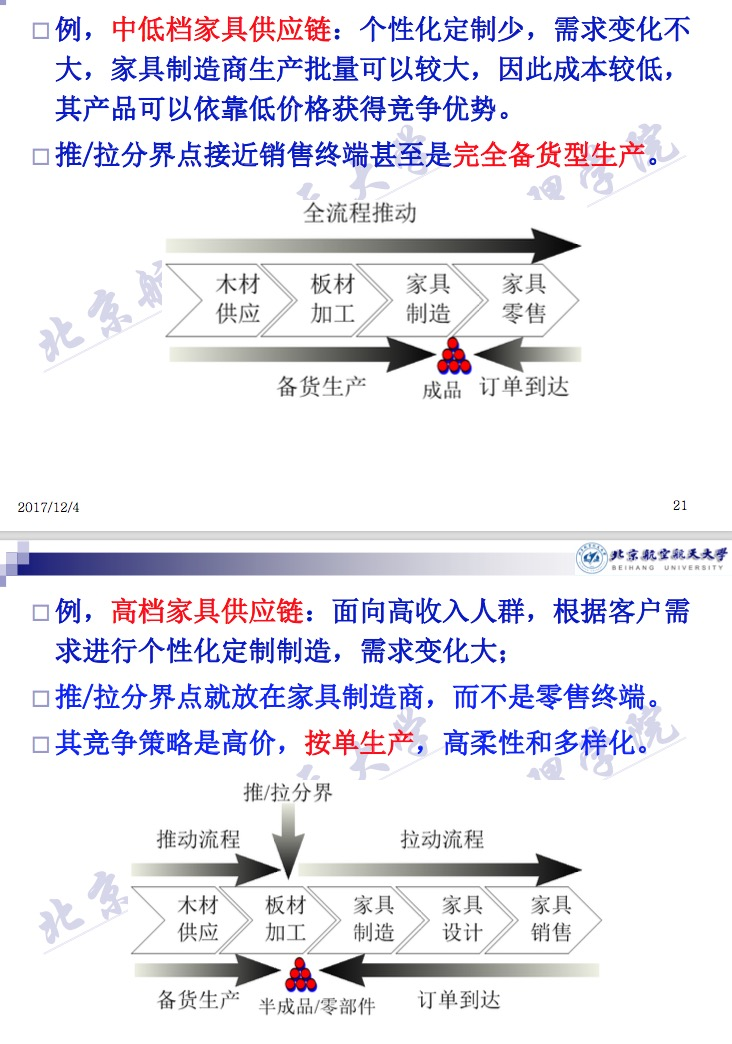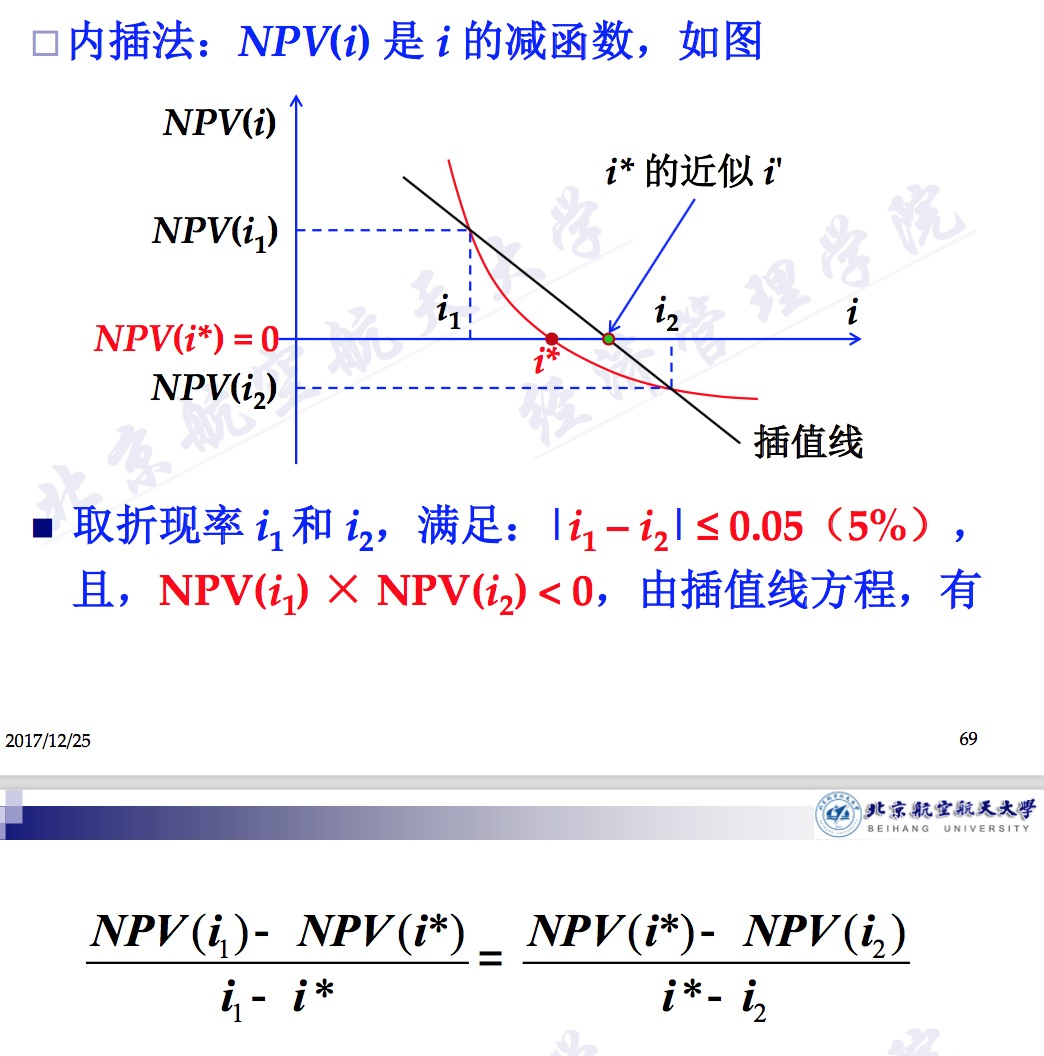# 经济管理浅学

## 经济管理重要概念

“牛鞭效应”：客户需求的微小波动，会引起上游

## 关于产业链与供应链## 经济可行性分析

0的n值。即$\sum_{t=0}^{n}F_t=0$ 的n值。其中$F_t$ 为时间点t的净现金流。

$$\sum_{t=0}^{n_d}F_t(1+i_0)^{-t} = 0$$

$$NPV = \sum_{t=0}^{n}F_t(1+i_0)^{-t}$$

$$NPV = \sum_{t=0}^{n}F_t(1+i_0)^{-t} = 0$$